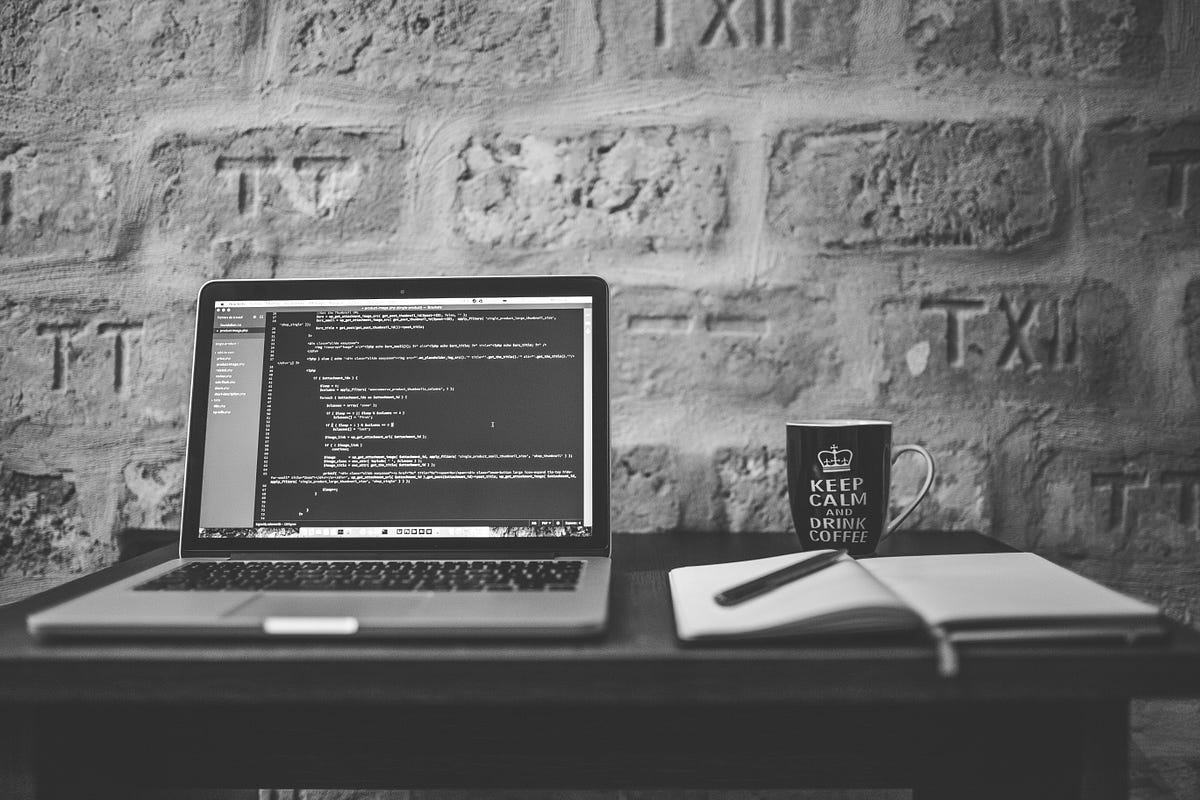# Data Visualization using Pandas, NumPy and Matplotlib Python LibrariesData Visualization using Pandas, NumPy, and Matplotlib Python Libraries. Numpy is a library for scientific computing in Python and also a basis for pandas.

To analyze which students secured the highest percentage in subjects like mathematics, physics, and chemistry we require a bar graph to display it. There are many ways to explore datasets. But in my point of view, Python plays a major role. It can be understandable with ease and requires fewer lines of code.

Why Build Visuals?

__ To communicate Data clearly and for exploratory data analysis

__ To share unbiased representation of data

__ Can use them to support recommendations to different stakeholders.

When creating a visual, always remember:

__ Any feature or design you include in your plot to make it more appealing and should hold up the message that the plot is meant to get across and not distract from it. It should be effective.

Before going to explore datasets, let us know about Pandas, NumPy, and Matplotlib.

## Python for Data Science - Learn Python, Pandas, NumPy, Matplotlib

Python tutorial for Data Science - Learn Python, Pandas, NumPy, Matplotlib, will take you from knowing nothing about Python to coding and analyzing data with Python using tools like Pandas, NumPy, and matplotlib. This is a hands-on course and you will practice everything you learn step-by-step.

## Data Visualization With Python: Matplotlib

Data visualization is the graphical representation of data in a graph, chart or other visual formats. It shows relationships of the data with images.

## Coding with Python, Pandas, Numpy & Matplotlib: Become a Data Scientist in 3 Hours | Part 2

Welcome to Part 2 of Become a Data Scientist! Today we go over the Python programming language, along with its central data science libraries: Pandas, NumPy, and Matplotlib. We describe Pandas Dataframes, NumPy n-dimensional arrays, and show off how to perform graphing both in Pandas and Matplotlib. We use Google Colab Notebooks, an interactive environment for writing Python code where we don't have to worry about any system dependencies!

## Python Data Science – A Free 12-Hour Course for Beginners. Learn Pandas, NumPy, Matplotlib

This free 12-hour Python Data Science course will take you from knowing nothing about Python to being able to analyze data. You'll learn basic Python, along with powerful tools like Pandas, NumPy, and Matplotlib. This is a hands-on course and you will practice everything you learn step-by-step. This course includes a full codebase for your reference [https://github.com/datapublishings/Course-python-data-science]. It kicks off with a one-hour introduction to basic programming concepts, proble

## Data Analysis with Python Course - Numpy, Pandas, Data Visualization

Learn the basics of Python, Numpy, Pandas, Data Visualization, and Exploratory Data Analysis in this course for beginners. This was originally presented as a live course.